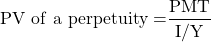Concept 1: Calculating PV and FV of Different Cash Flows | IFT World
101 Concepts for the Level I Exam

# Concept 1: Calculating PV and FV of Different Cash FlowsPresent value is the current value of a future cash flow.

• Longer the time period till the future amount is received, lower the present value.
• Higher the discount rate, lower the present value.

Future value is the value to which an investment will grow after one or more compounding periods.

• Longer the time period till which the investment is allowed to grow, higher the future value.
• Higher the interest rate, the higher the future value.

The future value and the present value of a single sum of money can be calculated by using the formulae given below or by using the TVM keys on a financial calculator (recommended approach for the exams).

FV = PV (1 + I/Y)N

PV = FV / (1 + I/Y)N

You invest U$100 today at an interest rate of 10% for 5 years. How much will you receive after five years? Solution: Plug the following values in the calculator. N = 5; I/Y = 10; PV = 100, PMT = 0; CPT FV =$161.05

An ordinary annuity is series of finite but equal cash flows which occur at the end of each period.

How much should you invest today at an interest rate of 10% to receive $100 at the end of each year for 5 years? Using the calculator: N = 5; I/Y = 10; PMT = 100; FV = 0; CPT PV =$379.08

An annuity due is a series of finite but equal cash flows which occur at the start of each period.

How much should you invest today at an interest rate of 10% to receive $100 at the beginning of each year for 5 years? Solution: Put the calculator in BGN mode and plug the following values. (Remember to exit the BGN mode once you are done with your calculations.) N = 5; I/Y = 10; PMT = 100, FV = 0; CPT PV =$416.98

perpetuity is a series of equal cash flows at regular intervals occurring forever. The present value of perpetuity can be calculated as:How much should you invest today at an interest rate of 10% to receive $100 at the end of each year forever? Solution: PV = 100/0.1 =$1,000

The present (future) value of any series of cash flows is equal to the sum of the present (future) values of the individual cash flows.

What is the future value of the following series of cash flows, given an interest rate of 10%?

$1,000 at the end of year 1,$2,000 at the end of year 2, $3,000 at the end of year 3,$4,000 at the end of year 4 and $5,000 at the end of year 5. Solution: The future value is 5000 + 4000 x 1.1 + 3000 x 1.12 + 2000 x 1.13 + 1000 x 1.14 =$17,156